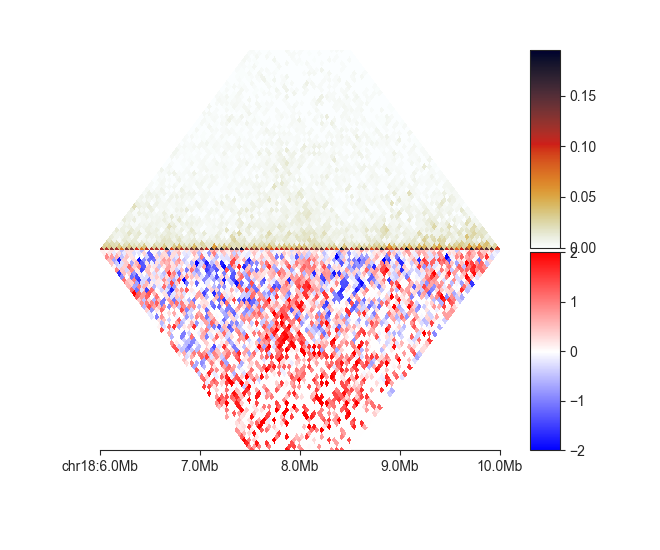# Matrix panel types¶

This section will provide details about the different types of panels in the command-line interface of `fancplot`.

## triangular¶

This is the common triangular heatmap plot used primarily for Hi-C data, but which is compatible with all sort of input matrices, including difference and fold-change comparisons. You simply provide it with a FAN-C compatible matrix file:

```fancplot chr18:6mb-10mb -p triangular output/hic/binned/fanc_example_50kb.hic
```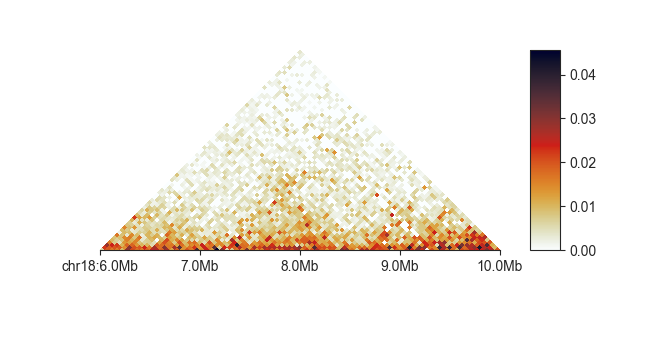You often need to adjust the matrix saturation, to highlight different parts of the image. You can do this interactively with `-r`:

```fancplot chr18:6mb-10mb -p triangular -r output/hic/binned/fanc_example_50kb.hic
```Or by explicitly setting a maximum saturation using `-vmax`, in this case to `0.05`.

You can truncate the triangle at a certain distance with `-m` for more compact plots:

```fancplot chr18:6mb-10mb -p triangular -m 2mb -vmax 0.05 output/hic/binned/fanc_example_50kb.hic
```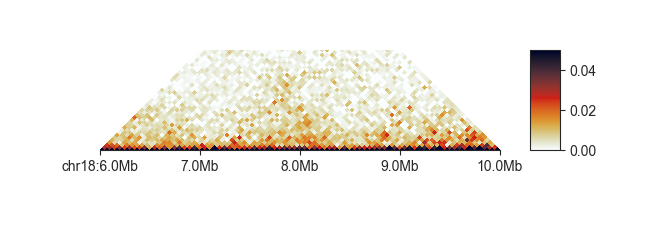You can also set the lower saturation values directly using `-vmin`:

```fancplot chr18:6mb-10mb -p triangular -vmin 0 -vmax 0.03 output/hic/binned/fanc_example_50kb.hic
```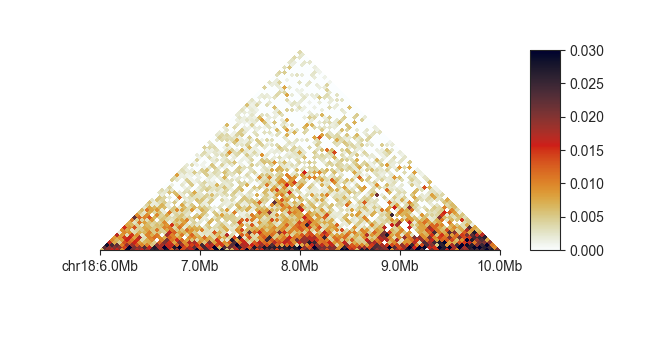To change the default colormap use `-c <matplotlib colormap>` - any colormap in matplotlib is supported.

```fancplot chr18:6mb-10mb -p triangular -c Greens -vmax 0.05 output/hic/binned/fanc_example_50kb.hic
```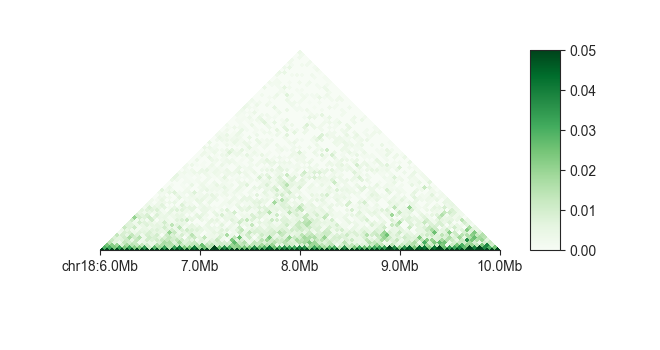You can change the color scale from linear to log using `-l`:

```fancplot chr18:6mb-10mb -p triangular -l -vmax 0.05 output/hic/binned/fanc_example_50kb.hic
```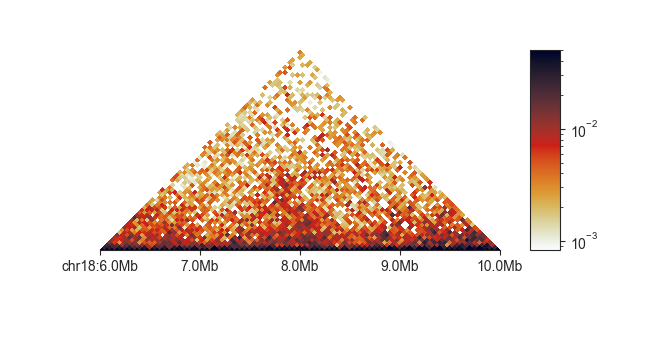By default, `triangular` plots normalised matrix data. You can turn this off on the fly to plot uncorrected data using `-u`:

```fancplot chr18:6mb-10mb -p triangular -u -vmax 30 output/hic/binned/fanc_example_50kb.hic
```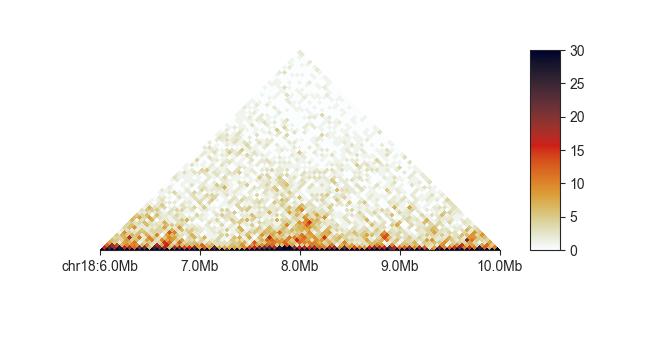Similarly, you can convert the matrix on the fly to an observed/expected (O/E) matrix using `-e`. When using this option, the matrix values are automatically log-2 transformed and the colorscale adjusts to a divergent colormap symmetrical around 0.

```fancplot chr18:6mb-10mb -p triangular -e -vmin -3 -vmax 3 output/hic/binned/fanc_example_50kb.hic
```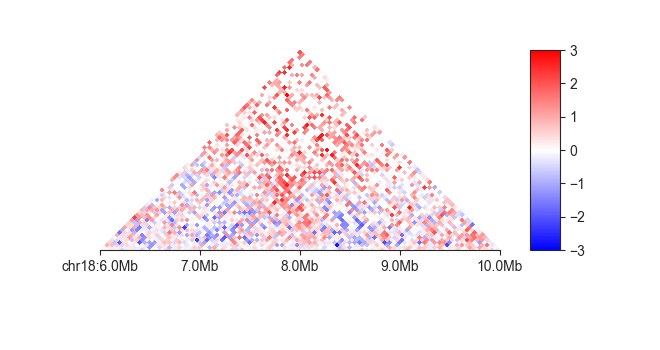## square¶

This plot is the common square matrix plot. It has nearly the same options as `triangle` and uses the same syntax:

```fancplot chr18:6mb-10mb -p square -vmax 0.05 output/hic/binned/fanc_example_50kb.hic
```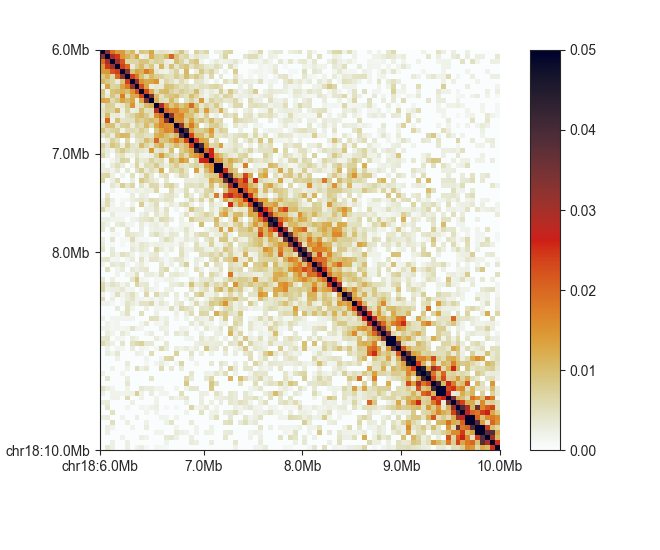In addition to `triangle`, `square` supports flipping the matrix upside-down with `-f`:

```fancplot chr18:6mb-10mb -p square -f output/hic/binned/fanc_example_50kb.hic
```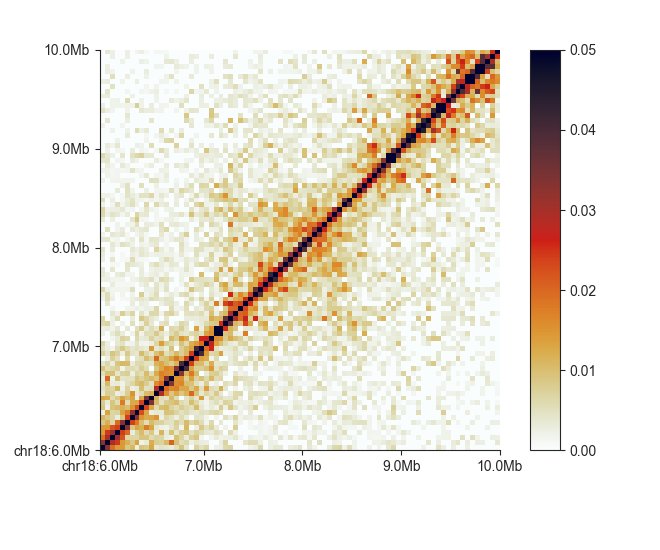## split¶

The `split` plot divides a heatmap along the diagonal to compare two matrices. `split` requires those matrices to be binned at the same resolution (or have identical region definitions), as they are combined into a single merged matrix on the fly. Besides providing two input matrices, one for the top and one for the bottom half of the heatmap, the plot works in the same way as `square`.

The plot assumes that the matrices are directly comparable and have been normalised to the same sequencing depth. If this is not the case, you can scale the matrices using the `-s` parameter, but note that this could potentially take a very long time.

## mirror¶

The `mirror` plot is built from two triangular heatmaps, the bottom one is flipped upside-down to give the effect of a reflection along the horizontal axis. This can be used to compare different matrices, including the same Hi-C dataset binned at different resolutions:

```fancplot chr18:6mb-10mb -p mirror -d 3mb output/hic/binned/fanc_example_50kb.hic output/hic/binned/fanc_example_100kb.hic
```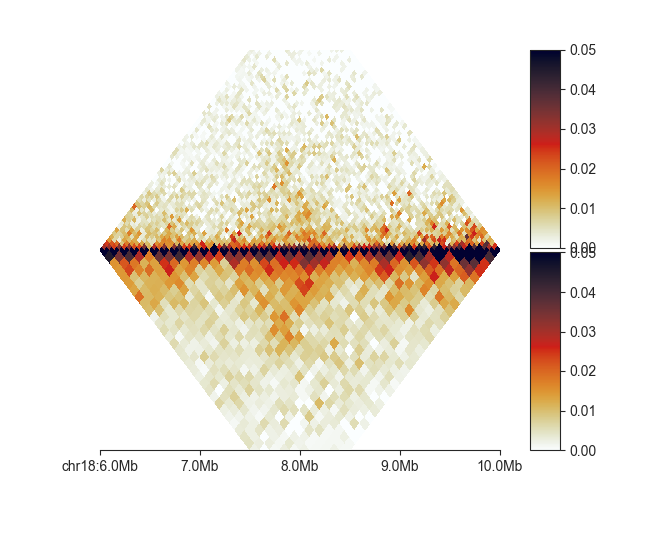As this plot essentially consists of two `triangular` plots stapled together, the parameters for the top and bottom plot can also be controlled independently. The parameter names match those of the `triangular` plot, but with the `u` prefix for the upper and `l` prefix for the lower plot: `--uvmin`, `--uvmax`, `--uc`, `--ue` and `--lvmin`, `--lvmax`, `--lc`, `--le`.

Here is one more example showing a matrix and its O/E transformed version:

```fancplot chr18:6mb-10mb -p mirror -d 3mb -le -lvmin -2 -lvmax 2 output/hic/binned/fanc_example_50kb.hic output/hic/binned/fanc_example_50kb.hic
```